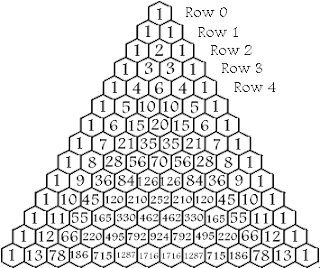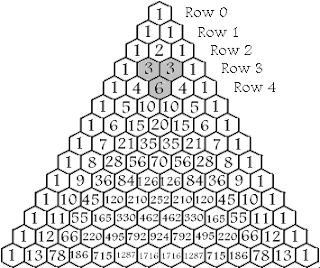## Wednesday, November 25, 2009

### SICP Exercise 1.12: Pascal's Triangle

From SICP section 1.2.2 Tree Recursion

Exercise 1.12 asks us to write a procedure that computes the elements of Pascal's triangle by means of a recursive process.The numbers along the left and right edges of the triangle are all 1, and each number inside the triangle is the sum of the two numbers directly above it.

We'll design our procedure to take the row and column numbers as arguments, and return the value of the element in that position.
(pascal row col)

We start counting both rows and columns at 0, as pictured in the diagram. So the procedure call
(pascal 4 2)
should return a value of 6, since that is the value in the 4th row and 2nd column.

The first thing we should note is that the nth row of the triangle contains exactly n columns. This means that we shouldn't expect to call our procedure with a column argument greater than the row argument. So, the following call would be expected to return nonsense information:
(pascal 3 4)

We should also note that the 0th and nth column of any row should return 1. This is enough to get us started.
(define (pascal row col)   (cond ((< row col) #f)         ((or (= 0 col) (= row col)) 1)         (else #t)))

Here we're just defining the initial logic of the procedure. If the row argument is less than the column, we simply return #f (probably not the best error message I've ever written, but it's not the focus of the exercise). If the column is the 0th or nth column, we return 1, otherwise we return a value of #t. This last value is just a placeholder which we'll need to replace with a calculation of the correct Pascal number.

To finish up the procedure, we need to recursively calculate values from the interior of the triangle. An interior value is defined as the sum of the two values directly above in the triangle. So, for example, to calculate Pascal(4, 2) we would sum Pascal(3, 1) and Pascal(3, 2). Each of these values (since there are both interior values) would be calculated in the same manner, but the recursive property of our procedure will take care of that for us.The two values to sum in order to calculate Pascal(row, column) are always going to be the value in the previous row and same column, Pascal(row-1, column), and the value in the previous row and previous column, Pascal(row-1, column-1). Translating this information to Scheme and adding it to our earlier procedure, we get our final answer to the exercise:
(define (pascal row col)   (cond ((< row col) #f)         ((or (= 0 col) (= row col)) 1)         (else (+ (pascal (- row 1) col)                  (pascal (- row 1) (- col 1))))))

Try several examples from Pascal's triangle illustration to verify that the results are correct. Note that since this procedure defines a recursive process, calculating larger values can be extremely time consuming. It takes a noticeable amount of time (a few seconds) on my machine to calculate values in the 25th row.

For more fun with Pascal's triangle see Pascal's Triangle and its Patterns.

Related:

For links to all of the SICP lecture notes and exercises that I've done so far, see The SICP Challenge.

sethreno said...

Good explanation.

I thought the exercise description was kinda vague: "Write a procedure that computes elements of Pascal's triangle by means of a recursive process."

I (mis)interpreted this to mean: "Given a depth compute the sum of all element values."

(define (pascal n)
(if (= n 0) n
(+ (* (pascal (- n 1)) 2) 1)))

Bill the Lizard said...

sethreno,
"a procedure that computes elements of Pascal's triangle" is a little ambiguous. If I were teaching a course using SICP, I'd accept that interpretation of the question.

However, your solution is off by one.

> (pascal 0)
0
> (pascal 1)
1
> (pascal 2)
3
> (pascal 3)
7
> (pascal 4)
15

If you want the sum of all the elements in the nth row of Pascal's triangle, just raise 2 to the nth power.Anonymous said...

I was returning 0 if the column is greater than the row so I would get correct result for correct input and 0 for incorrect input. Returning #f is probably a better option since 0 is a response and calling the procedure with a column greater than the row should probably not give a response. Once again, very useful post.

Фёдор Ананьев said...

Oh thank you. Solved this exercise myself before and managed to do it in three times more lines. What a shame.

tokland said...

In fact values "outside" the triangle are zeros, no need to return an special value.Anonymous said...

Hey Bill,
I recently discovered your blog and I love it.
I recently started reading SICP and your solutions and explanations are a great help.
I also love your lecture notes.
I don't know if you already knew this but there is another (iterative) way to calculate the elements of the Pascal's triangle.
I give my code below.

(define (pascal2 r c)
(define (iter col val)
(if (= col c)
(/ (* val (- (+ r 1) col)) col)
(iter (+ col 1)
(/ (* val (- (+ r 1) col)) col))))
(cond ((or (< c 0) (> c r)) 0)
((or (= c 0) (= c r)) 1)
(else (iter 1 1))))

I found this on Wikipedia. Here's the link.
http://en.wikipedia.org/wiki/Pascal%27s_triangle#Calculating_an_individual_row_or_diagonal_by_itself_.28Gray.27s_Theory.29Anonymous said...

The elements of the Pascal's triangle are the Binomial coefficients, i.e., they are the coefficients of the terms in the expansion of (x + y)^n, where n is a natural number.
Since the coefficients of the terms not in the expansion are zero, the values outside the Pascal's triangle should be 0 not #f.

Jan Herich said...

Slightly different solution:

(define (pascal-elem row col)
(cond ((or (< col 0) (> col row)) 0)
((< row 2) 1)
(else (+ (pascal-elem (- row 1) (- col 1))
(pascal-elem (- row 1) col)))))

Dhruv Dave said...

I know a little bit of clojure, so anytime I make attempt at SICP exercise, I do it in clojure first and translate the code into scheme.
So my question is, would you ever consider this solution?

(define (dec number)
(- number 1))

(define (inc number)
(+ number 1))

(define (drop n coll)
(if (and (not (null? coll))
(not (= 0 n)))
(drop (dec n) (cdr coll))
coll))

(define (take n coll)
(if (and (not (null? coll))
(not (= 0 n)))
(cons (car coll) (take (dec n) (cdr coll)))
'()))

(define (count coll)
(if (null? coll)
0
(inc (count (cdr coll)))))

(define (pn coll n step)
"Partition the collection. 'n' is the number of elements in a
partition and 'step' is the offset."
(if (and (not (null? coll))
(> (count coll) step))
(cons (take n coll) (pn (drop step coll) n step))
'()))

(define (pascal-triangle n)
"When supplied with a row number, return a list of numbers corresponding to pascal's triangle.
Row number starts from '0'."
(cond
((= n 0) '(1))
((= n 1) '(1 1))
((<= 2 n) (cons 1
(reverse
(cons 1
(map (lambda (s) (apply + s))
(pn (pascal-triangle (- n 1)) 2 1))))))))

;; how it works
1]=> (pn '(1 3 3 1) 2 1)

;Value 38: ((1 3) (3 3) (3 1))

1 ]=>

1 ]=> (pascal-triangle 0)

;Value 39: (1)

1 ]=> (pascal-triangle 1)

;Value 40: (1 1)

1 ]=> (pascal-triangle 2)

;Value 41: (1 2 1)

1 ]=> (pascal-triangle 3)

;Value 42: (1 3 3 1)

1 ]=> (pascal-triangle 4)

;Value 43: (1 4 6 4 1)

1 ]=> (pascal-triangle 5)

;Value 44: (1 5 10 10 5 1)

1 ]=> (pascal-triangle 6)

;Value 45: (1 6 15 20 15 6 1)

1 ]=>

Dylan Beckwith said...

Seeing as "Pascal's Triangle" is a listed representation of the binomial coefficients.
i defined and ran a (choose n k) procedure thru a coefficient procedure.
Both Algorithmically and Mathematically, i believe this to be the strongest solution.. Enjoy!

(define (bi-co col row)

(define (choose n k)
(/(fact n)
(* (fact (- n k))
(fact k))))

(define (fact x)
(define(fact-iter x product)
(if(zero? x)product
(fact-iter (sub1 x)
(* product x))))
(fact-iter x 1))Anonymous said...

I loved the format of Pascal's Triangle. Just wanted to let you know that the fourteen row has an error.Anonymous said...

I though the point was to build the actual triangle:

;; build a triangle n-levels deep
;; pascals-triangle :: int -> [[int]]
(define (pascals-triangle n)
;; reduce :: (a -> a -> a) -> [a] -> [a]
(define (reduce f l)
(cond ((null? l) '())
((= (length l) 1) l)
(else (cons (+ (car l) (cadr l))
(reduce f (cdr l))))))
(define (loop l)
(cond ((< n (length l)) l)
(else (let ((last (cons 0 (car l))))
(loop (cons (reduce + last) l))))))
(loop '((1))))

> (pascals-triangle 10)

(1 10 45 120 210 252 210 120 45 10 1)
(1 9 36 84 126 126 84 36 9 1)
(1 8 28 56 70 56 28 8 1)
(1 7 21 35 35 21 7 1)
(1 6 15 20 15 6 1)
(1 5 10 10 5 1)
(1 4 6 4 1)
(1 3 3 1)
(1 2 1)
(1 1)
(1)